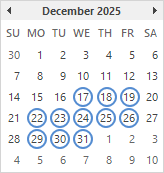# Days between Dates

To calculate the number of days between two dates in Excel, subtract the start date from the end date, use DAYS or use DATEDIF.

1. Subtract the start date (A1) from the end date (A2).2. Use the DAYS function in Excel 2013 or later. The first argument is the end date. The second argument is the start date.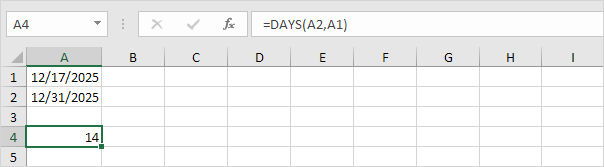3. Use the DATEDIF function. The first argument is the start date. The second argument is the end date. Fill in "d" for the third argument to get the number of days between two dates.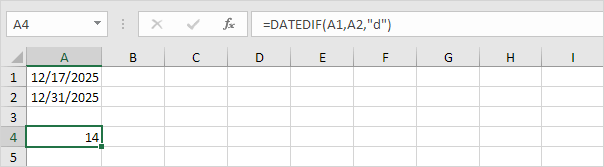Tip: visit our page about the DATEDIF function to find out how to get the number of weeks, months or years between two dates in Excel.

4. The formula below returns the same number of days. Use the DATE function in Excel to hardcode a date.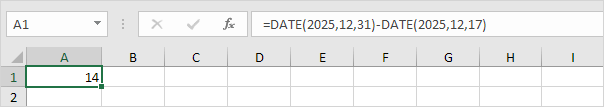Explanation: the DATE function accepts three arguments: year, month and day.

5. Bonus. Use the NETWORKDAYS function to return the number of workdays between two dates in Excel. NETWORKDAYS excludes weekends (Saturday and Sunday).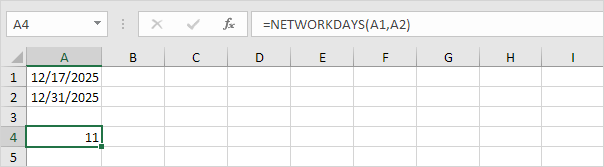Explanation: the NETWORKDAYS function includes the start date and the end date. The calendar below helps you understand this formula. Simply count the number of circles.